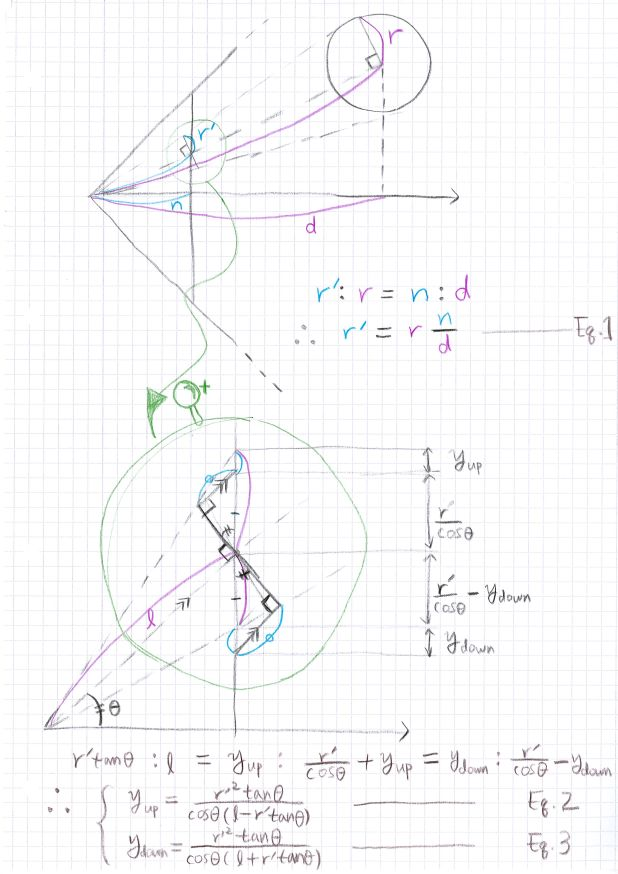Recently I had a chance to implement a toy ray-tracer for a scene with spheres only. Once a brute-force approach had been coded, I tried to optimize it by pre-sorting spheres into screen tiles(i.e. a uniform grid on the near view plane) so that only spheres binned to the tile can be considered when tracing the ray for a pixel in that tile. This wasn’t that simple as I originally had expected because in a perspective projection a sphere projected to the 2D screen is not a  circle(in fact, not a shape representable by any simple equation at all, AFAIK). But, with a little bit of geometry and trigonometry gimmickry, it was possible to compute a tight rectangular bound of the projected sphere so that it can be binned to all relevant tiles.

The picture below, which manually drawn by me, shows the situation and geometric parameters involved. Definitely not a fine art, but this was the best possible with my drawing skill :)Geometry of a 'sphere in a frustum projected to the near plane'

As you can see, this shows only the situation in the vertical(y) dimension, but the same derivation goes for the horizontal(x) dimension, also. A sphere of radius r is being projected here. The downscaled radius, r’ at the near plane can be easily calculated using a proportional expression(Eq. 1). In the zoomed-in diagram at the bottom, you can see how the final extent in y dimension should be arranged. In the end, it will be like:

r’/cos(theta)-Ydown < (Y – projected_sphere_center) < r’/cos(theta) +Yup

Here, the projected_sphere_center means the projection of the sphere center to the near plane. the theta is an angle between the view vector and the vector from eye to the projected sphere center. To get the Yup & Ydown, two proportional relations used again, one for the up part, the other for the down part(refer to the blue/purple arcs in the zoom-in part). I hope the proportional relations are obvious from my hand-drawing. Once Yup and Ydown calculated using Eq.2, Eq. 3, the extent of Y is obtained like the inequality above(note that with the convention of positive theta in a counter-clock-wise rotation, the same pair of equations can be used to get the extent even when the sphere is below the view vector rather than above). One can get the extent of X in the same way, and with this 2D extent obtained, screen tiles which overlap with this rectangular area can be easily identified.

In hindsight, the result is quite basic and requires only the primitive knowledge of geometry & trigonometry. Nonetheless I thought it would be meaningful to document the derivation. I hope this is useful for some people. If you find any error or happen to know a better way, please enlighten me!